April 17, 2020

## How-To use a csv file as filter and destination path in LimagitoX File Mover

Another example of how Pascal Script can be used. In this how-to we’ll explain you how the content of a csv file can be used to filter the source file and at the same time this csv file will be used to set the destination path.

In this example we use C:\Test\Demo as base folder. It contains 3 subfolders which will be used by the Moving Rule.

1. C:\Test\Demo\Csv  :  contains the Csv file which we will scan for its content. The content will give us the file filter and destination path.
2. C:\Test\Demo\In  :  folder will be used as source of the moving rule
3. C:\Test\Demo\Out  :  folder will be used as destination path. We’ll receive this info from the csv file.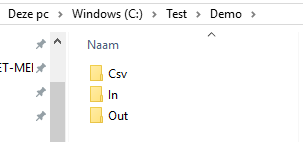In the Csv subfolder we created an excel file. First column contains the filename filter. We’ll search for files which contain this string in its filename. The second column is used for the destination path when this file is found.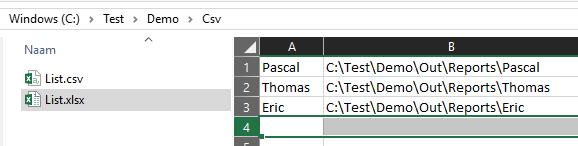Next we did an export of this excel file to csv. We need this cvs file for our Pascal Script.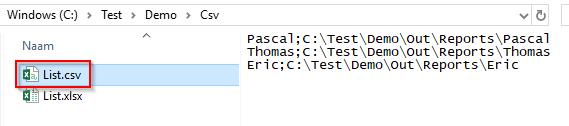Content of the Source Folder: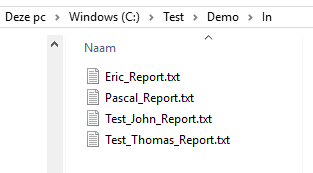Select this folder in the Source Setup (WIN).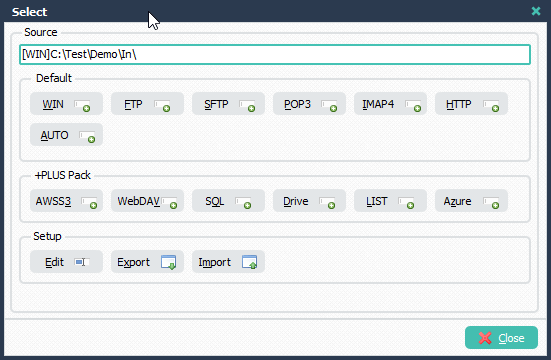Setup of the File Filter. Important, select RegEx Include filter and add %VSB as filter.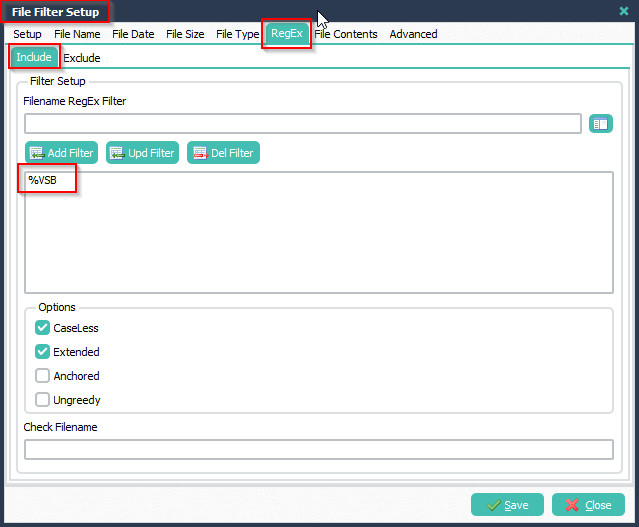Open the Pascal Script Setup: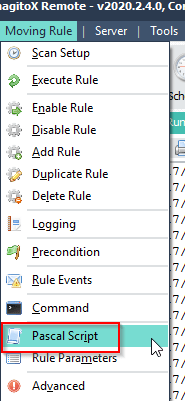Add the following ‘On Rule Begin’ Pascal Script. Don’t forget to adjust the ctCsvFile constant (depends on your setup).

```Var
iList: Integer;
tmpList: TStringList;
tmpEntry: String;
tmpName: String;
tmpCount: Integer;
tmpPos: Integer;
Const
ctCsvFile = 'C:\Test\Demo\Csv\List.csv';
ctCsvSep = ';';
Begin
psExitCode:= 0;
psVSA := '';
psVSB := '';
Try
tmpList := TStringList.Create;
Try
If tmpList.Count > 0 Then
Begin
tmpCount := tmpList.Count - 1;
For iList := 0 to tmpCount Do
Begin
tmpEntry := Trim(tmpList.Strings[iList]);
tmpPos := Pos(ctCsvSep, tmpEntry);
If tmpPos <> 0 Then
Begin
tmpName := Copy(tmpEntry, 1, tmpPos-1);
If iList = tmpCount Then
psVSB := psVSB + '.*' + tmpName + '.*'
Else
psVSB := psVSB + '.*' + tmpName + '.*|'
End;
End;
psVSA := tmpList.CommaText;
// Debug Info
psLogWrite(1, '', 'psVSA: ' + psVSA);
psLogWrite(1, '', 'psVSB: ' + psVSB);
// Set psExitCode
If (psVSA <> '') And (psVSB <> '') Then
psExitCode := 1;
End;
Except
psLogWrite(1, '', 'Load Script File Exception');
End;
Finally
tmpList.Free;
End;
End.```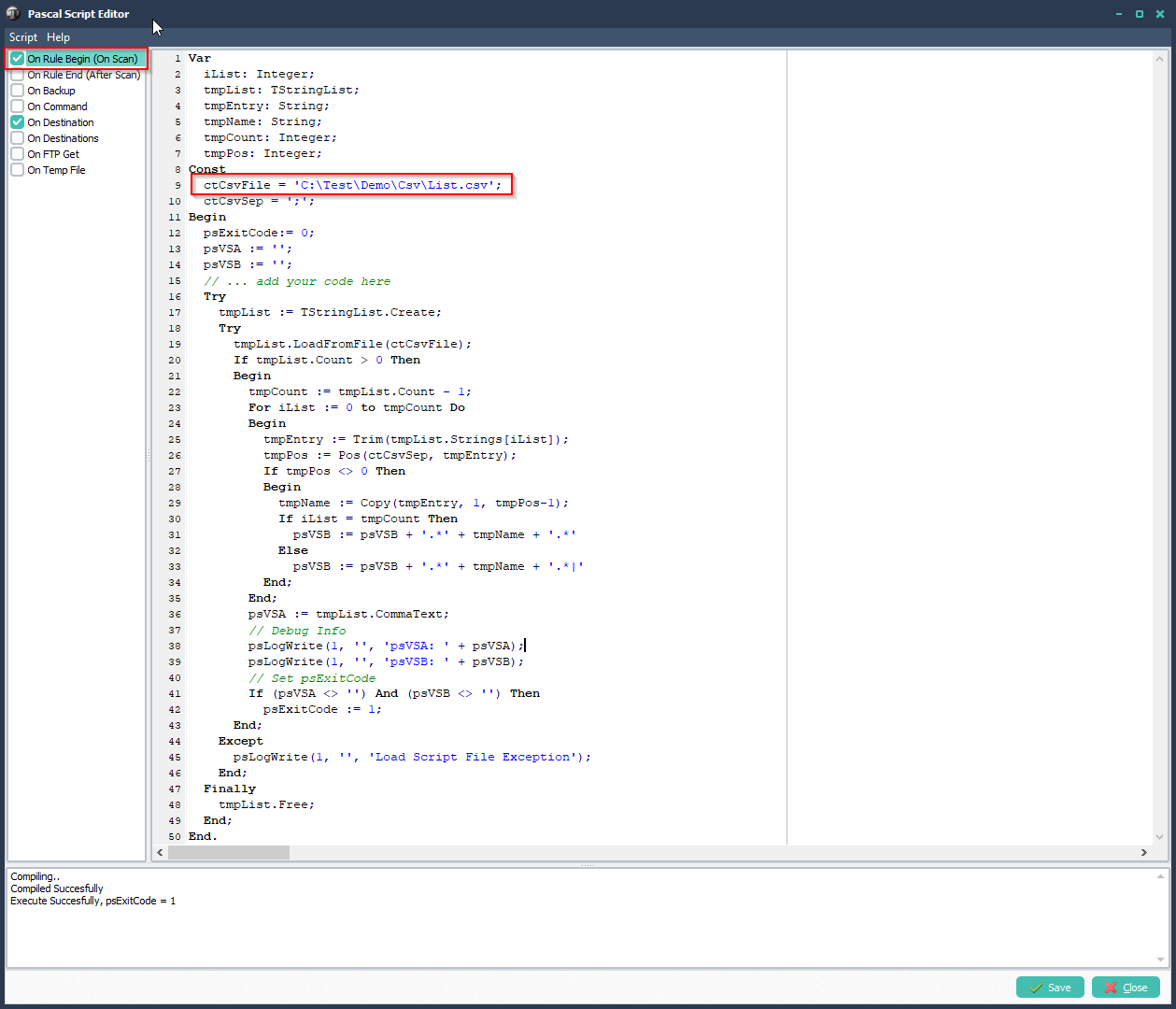Add the following ‘On Destination’ Pascal Script.

```Var
iList: Integer;
tmpList: TStringList;
tmpEntry: String;
tmpName: String;
tmpPath: String;
tmpCount: Integer;
tmpPos: Integer;
Const
ctCsvSep = ';';
Begin
psExitCode:= 0;
psVSC := '';
Try
tmpList := TStringList.Create;
Try
tmpList.CommaText := psVSA;
If tmpList.Count > 0 Then
Begin
tmpCount := tmpList.Count - 1;
For iList := 0 to tmpCount Do
Begin
tmpEntry := Trim(tmpList.Strings[iList]);
tmpPos := Pos(ctCsvSep, tmpEntry);
If tmpPos <> 0 Then
Begin
tmpName := Copy(tmpEntry, 1, tmpPos-1);
If Pos(tmpName, psFileName) <> 0 Then
begin
tmpPath := Copy(tmpEntry, tmpPos+1, length(tmpEntry)-tmpPos);
If tmpPath <> '' Then
Begin
psVSC := tmpPath;
Break;
End;
End;
End;
End;
// Debug Info
psLogWrite(1, '', 'psVSC: ' + psVSC);
// Set psExitCode
If (psVSC <> '') Then
psExitCode := 1
Else
psLogWrite(1, '', 'Could not find a path for: ' + psFileName);
End;
Except
psLogWrite(1, '', 'Find Destination Path Exception');
End;
Finally
tmpList.Free;
End;
End.```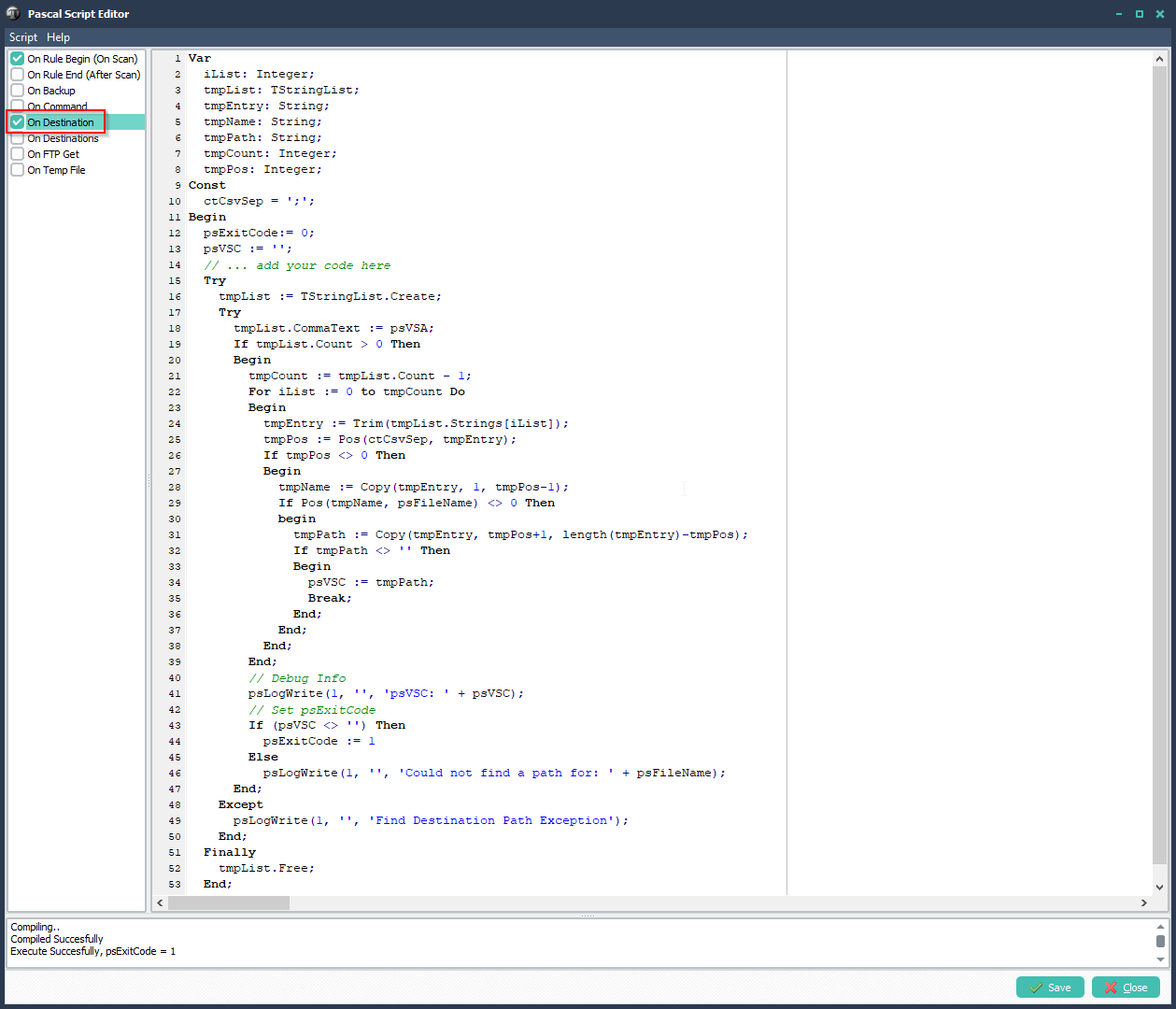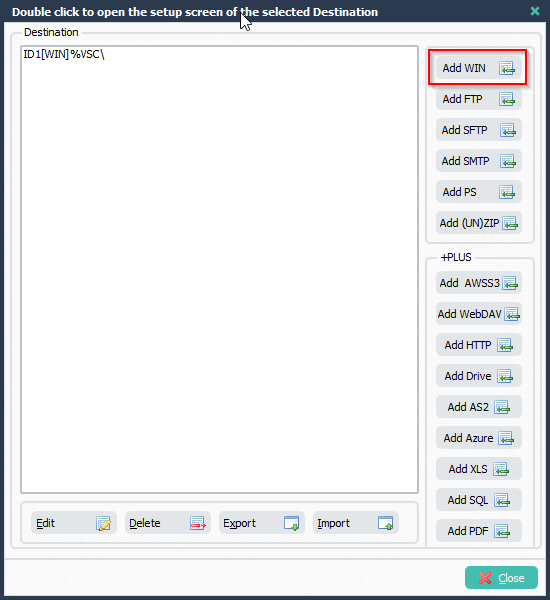Set ‘Select Directory’ to %VSC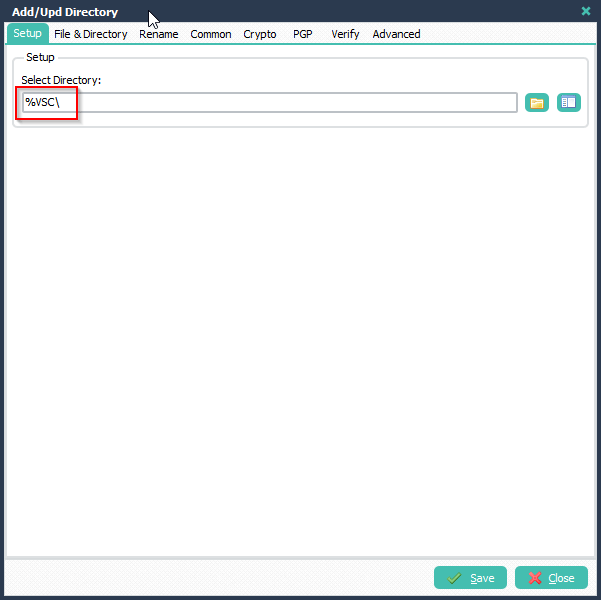Enable and trigger the rule. The Source files will be filtered and moved to the destination as set in the csv file.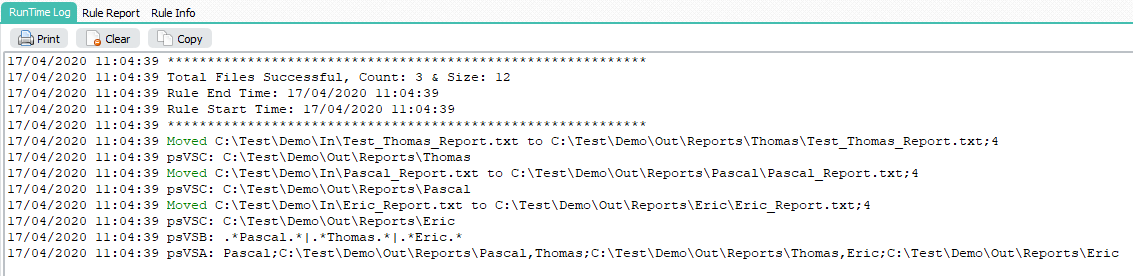Destinations created with information from the csv file: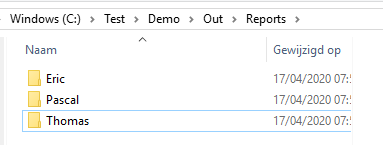Destination content example: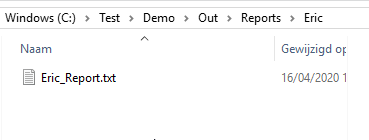This is just an example of what can be done using Pascal Script. If you need any help using Pascal Script, please let us know.

Regards,

Limagito Team

By Limagito-Team Share: